## Algebra

Showing posts with label polynomial. Show all posts
Showing posts with label polynomial. Show all posts

### Synthetic Division

When dividing polynomials of the form p(x)/(x-a) we can use synthetic division as a shortcut for polynomial long division. Below we divide using traditional polynomial long division and synthetic division side by side.

Divide.
Both processes give the same result, x^2 - 3x - 2. However, synthetic division uses only the coefficients and requires much less writing.  To understand synthetic division, we walk you through the process below. Be sure the polynomials are in standard form, that is, each term is arranged in descending order from highest power to lowest.

Step 1: Write the root a determined from (x-a) and the coefficients of the polynomial in the first line.
Step 2: Bring down the first coefficient and we are ready to begin.
Step 3: Multiply a by the first coefficient and write the result under the second coefficient.
Step 4: Add the second column and write the result below.
Step 5: Repeat the process for all of the remaining columns.
Step 6: The numbers along the bottom are the coefficients of the result in standard form beginning with a term of degree one less than the original polynomial. The last number is the remainder.
To finish, clearly present the answer to your reader.  Next we do an example with a remainder. Just as we do in polynomial long division, we add a term that is the remainder over the divisor.

Divide.
Here the root (or zero) of (x+5)  is -5.
Multiplying both sides by the divisor (x+5) we have the following.
We mentioned that the polynomials are required to be in standard form. Sometimes there will be "missing terms."  That is, not all powers will have nonzero coefficients.  In this case, we use 0 as placeholders when performing synthetic division.

Divide.

Sometimes the root will be a fraction.

Divide.

It is interesting to note that the result has a GCF of 2 and we can do the following algebraic manipulations:
In short, this gives us a method of factoring a more complicated polynomial.

---

### Introduction to Factoring and Factor by Grouping

Factoring is one of the more important skills to learn in a beginning algebra course.

The idea is to work the distributive property in reverse. The goal is to write polynomials as products of simpler polynomials.To decide what the GCF (Greatest Common Factor) is, look for the largest factor that divides into all the terms. In other words, what common term will divide into all the given terms evenly?In the above example, the variable z is not common to all the terms so it is not included in the GCF. Use the smallest exponent for the common variables x and y.

Identify the GCF.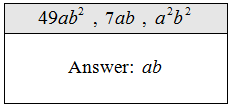Factor out the GCF:Step 1: Identify the GCF.Step 2: Divide each term by the GCF to determine the remaining factor.Step 3: Check by multiplying.The check is not really necessary; however, it is good practice to at least check the factoring mentally.

Factor the GCF out of the expression.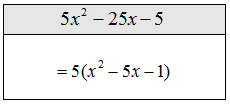Tip: Be careful to use a 1 when the entire term factors out. This is where a mental check is important. Be sure that if you were to distribute you would get back to the original expression.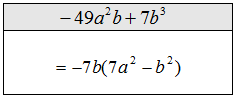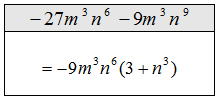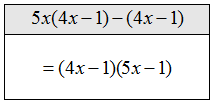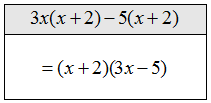All of the above problems require only one step. The hard part is to identify the GCF. Here is the check for the last solved problem.Factoring 4-Term Polynomials by Grouping
Now we will use the idea of factoring out the GCF in a technique called factoring by grouping of four-term polynomials. The steps are as follows.

Factor:Step 1: Group the first two terms and the last two terms.  Factor out the GCF of both groupings.Step 2: If the remaining binomial factors are the same factor it out.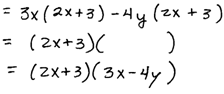Step 3: Check by multiplying.Factor by grouping.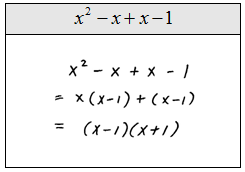Sometimes you will encounter 4-term polynomials where factoring by grouping does not seem to work.  When this is the case, you can try to rearrange the terms in a different order and try again. For example, try to factor the following polynomial  by grouping.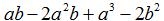Rearrange the terms and try again.You can check by multiplying.